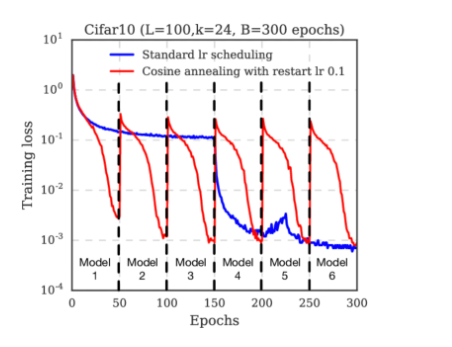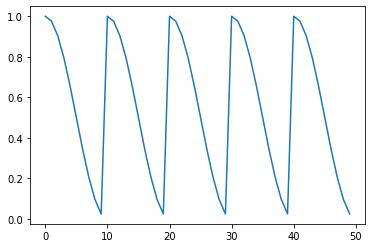# Cosine Learning Rate Decay

Hi, guys. I am trying to replicate the `torch.optim.lr_scheduler.CosineAnnealingLR`. Which looks like:However, if I implement the formula mentioned in the docs, which is:

It is simply up-moved cosine function, instead of the truncated one above.

``````import numpy as np
from matplotlib import pyplot as plt
import math
lmin=0.001
lmax=0.01
tmax=50
x=[i for i in range(200)]
y=[lmin+0.5*(lmax-lmin)*(1+math.cos(i*math.pi/tmax)) for i in range(200)]
for i in range(200):
if (i/tmax)%2==1:
y[i+1]=y[i]+0.5*(lmax-lmin)*(1-math.cos(1/tmax))
# pass
plt.plot(x,y)
``````I wonder if there’s anything wrong with my code?

You might want to use `CosineAnnealingWarmRestarts` as seen here:

``````optimizer = torch.optim.SGD([nn.Parameter(torch.randn(1, 1))], lr=1.)
T_0 = 10

scheduler = torch.optim.lr_scheduler.CosineAnnealingWarmRestarts(optimizer, T_0)

lrs = []
for epoch in range(50):
scheduler.step(epoch)
print(scheduler.get_last_lr())
lrs.append(scheduler.get_last_lr())

lrs = np.array(lrs)
plt.plot(lrs)
``````

Output:Thank you, Mr. Patrick. I finally figured out that `T_cur` represents the epochs since last restart, instead of the accumulated epochs. In my code,

``````y=[lmin+0.5*(lmax-lmin)*(1+math.cos(i*math.pi/tmax)) for i in range(200)]
``````

should be changed to

``````y=[lmin+0.5*(lmax-lmin)*(1+math.cos((i%tmax)*math.pi/tmax)) for i in range(200)]
``````

By the way, do you think it would be a good idea to gradually decay `eta_max` during training (maybe directly revert to the original `eta_max` might break the suboptimal to much)?

Oh, I don’t know as I’m not experienced enough with these learning rate scheduler schemes, so let’s wait for an expert to chime in.Thanks Sir for the reply and the help. I will try to carry out experiments to verify my conjectureAn interesting questions. I found a link online which describes chained learning rate schedulers. Maybe this can be used to realize a decaying `eta_max` by combining CosineWR with something like Exponential decay.